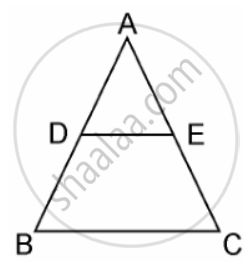# Using Converse of basic proportionality theorem, prove that the line joining the mid-points of any two sides of a triangle is parallel to the third side. (Recall that you have done it in Class IX). - Mathematics

Sum

Using Converse of basic proportionality theorem, prove that the line joining the mid-points of any two sides of a triangle is parallel to the third side. (Recall that you have done it in Class IX).

#### Solution

Given, In ΔABC

D and E are the midpoints of AB and AC respectively, such that,

AD = BD and AE = EC.We have to prove that: DE || BC.

Since, D is the midpoint of AB

⇒ (AD)/(BD)  = 1  .......(i)

Also given, E is the midpoint of AC.

∴ AE = EC

⇒ (AE)/(EC) = 1

From equation (i) and (ii), we get,

(AD)/(BD) = (AE)/(EC)

By converse of Basic Proportionality Theorem,

DE || BC

Hence, proved.

Concept: Basic Proportionality Theorem (Thales Theorem)
Is there an error in this question or solution?

#### APPEARS IN

NCERT Mathematics Exemplar Class 10
Chapter 6 Triangles
Exercise 6.2 | Q 8 | Page 129

Share